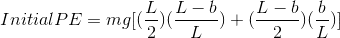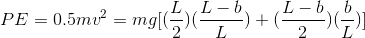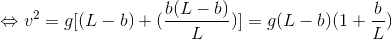# Finding time taken to fall off table

• Arctic

## Homework Statement

A uniform chain of length L lies on a smooth table with part of its length b initially hanging over the edge. Show that the time required for it to fall off the table when released from rest is given by

t = √(L/g) arccosh (L/b)

## Homework Equations

http://img10.imageshack.us/img10/2203/integral.png [Broken]

## The Attempt at a Solution

Not sure how to begin, since the acceleration of the chain keeps increasing as b increases. b is in turn related to the acceleration of the chain.

Last edited by a moderator:
Welcome to PF!

Hi Arctic! Welcome to PF!(have a square-root: √ and try using the X2 tag just above the Reply box)
A uniform chain of length L lies on a smooth table with part of its length b initially hanging over the edge. Show that the time required for it to fall off the table when released from rest is given by

t = (L/g)^0.5 arccosh (L/b)

Either use good ol' Newton's second law, or (slightly quicker, since it gives you a first-order equation) simply use conservation of energy.(But if you use conservation of energy, don't forget you have to integrate to find the PE)

Using conservation of energy I get:This is by breaking the chain into 2 parts: the part of length L-b lying on the table and the part b over the edge. I also take PE = 0 at height L/2 below the table to simplify calculations later

When the chain has fallen off the table, PE = 0 (since its center of gravity is at L/2 below the table) andNot sure how to continue from here, assuming this is right that is.

As for Newton's 2nd law..
F=ma
mgb/L = ma
a = gb/L
dv/dt = gb/L
d2b/dt2 = gb/L
dt2 = d2b L/gb

Again, I am stuck here